• Forums
• Posts
Latest Posts
Active Posts
Recently Visited
Search Results
• Page Extras
• Menu
• Forum Themes

### Behringer Deepmind 12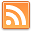Author
CedricLawdeMax Output Level: -90 dBFS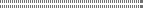• Total Posts : 11
• Joined: 2015/04/07 17:33:14
• Status: offline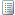2017/08/13 10:14:49 (permalink)

# Behringer Deepmind 12

Hello,
You will find below the text for the Instrument definitions for the Behringer Deepmind 12.
This is to be copied in a text file named "Behringer Deepmind 12.ins".
Have a good time!

; Cakewalk Instrument Definition
; For BEHRINGER DEEPMIND12
; V1.0c by Cedric Lawde 2017
;
; [V1.0c] Rename some CC controls.
; [V1.0b] Too long string NRPN name for Sonar truncated.
; [V1.0a] Initial release.
; [note 1] * are duplicates patches
; [note 2] First patch are initialy "Blue Dolphin BC".
;

; ----------------------------------------------------------------------

.Patch Names

[DM12 Bank A user]
0=1 Default Program
1=2 Chord Play RD*
2=3 Heaven Pad KA*
3=4 Rich Rises DK*
4=5 Hammer Bass KA*
5=6 JunoProximityMS*
6=7 Sweep & Fold RD*
7=8 Robbie2 RBr*
8=9 Outer Rim JD*
9=10 In My Spot BC*
10=11 TensionBass RD*
11=12 Bullish DG
12=13 Symphonia KA*
13=14 Mono Cloud DK*
14=15 Technodisco JD*
15=16 AfterGlow RD*
16=17 Brassy KA*
17=18 2Wheel Drive RB*
18=19 EventHorizen RD*
19=20 1973 RD*
20=21 MouseChord DK*
21=22 Phat Morph KA*
22=23 BlissPad RD*
23=24 Up In Clouds RD*
24=25 Dark Apostle JD*
25=26 Gianni'Arp SG*
26=27 Slasher DK*
27=28 ass Punch DK
28=29 TineEP1 DG
29=30 Massive KA*
30=31 BOC Pad RD*
31=32 Juppy Synth KA*
32=33 Helix Repeat RB*
33=34 HammondCrunchDG
34=35 Nightdrive BC*
35=36 Brass Pad BC*
36=37 Influx Pad KA*
37=38 Tundra RD*
38=39 CS Strings KA*
39=40 Nice Pad KA*
40=41 Echo Base JD*
41=42 Arptastic2 RB*
42=43 Movement ST*
43=44 Poly Dream RD*
44=45 Funkin'FilthyBC*
45=46 Battleground BC*
46=47 SynthBass 3 KA*
47=48 Ethereal Pad RD*
48=49 Flutter Seq DK*
49=50 In The Air 1 KA*
50=51 ModDecimator JD*
51=52 Rich Chords RD*
52=53 DeepEP4 BC*
53=54 Human Pad KA*
54=55 Chord Pulse RD*
55=56 Wide StringsST*
56=57 ReedEP1 DG
57=58 Abyss JD*
58=59 Pwm Pad RB*
59=60 Slow Glide RD*
60=61 Movement BC*
61=62 Punch Bass KA*
62=63 DreamSeq RD
63=64 Soft Mono DK*
64=65 ElecKoto DG*
65=66 Zero Bass JD*
66=67 Flead RB*
67=68 Planet Zion RD*
68=69 Arp+Seq BC*
69=70 Rock On KA*
70=71 Fairy Tales RD*
71=72 Lost Verb DK*
72=73 Bilitis 1 KA*
73=74 Groovy! ST*
74=75 GlassPoly RD*
75=76 Top Bass BC*
76=77 Funky Lead KA*
77=78 Sweet Pulse RD*
78=79 Soft Fifth DK*
79=80 Spaceman KA*
80=81 Chiffy Arp ST*
81=82 Alien Shift RD*
82=83 Mean Lead BC*
83=84 Rhythm Steps KA*
84=85 ReedEP3 DG*
85=86 PhaseClav DG*
86=87 Dream Pad KA*
87=88 Coil Soup ST*
88=89 2PSweepPad DG*
89=90 DeepCheez BC*
90=91 Cl&OpHats DG*
91=92 Warm BD DG*
92=93 Sub Monster ST*
93=94 Sync Lead RD*
94=95 Squarp BC*
95=96 Sawyer Lead KA*
96=97 Rez BD DG*
97=98 Berlin KA*
98=99 Latey Bass ST*
99=100 Rotary RhodesDG*
100=101 HerbieComp DG*
101=102 Iridescent BC*
102=103 Field Space RD*
103=104 Snr1 DG*
104=105 Flange Lead KA*
105=106 Six Oh OnesiDK*
106=107 MainBrass DG*
107=108 Magic Key RD*
108=109 DeEP2 BC*
109=110 Soft Swells DK*
110=111 KApro Pad KA*
111=112 Dream Arp JD*
112=113 Old School RD*
113=114 String Pad KA*
114=115 Polygroove BC*
115=116 TineEP2 DG*
116=117 ChordCrunch DK*
117=118 Miami Bass KA*
118=119 Jumpy! ST*
119=120 Juice3DG*
120=121 Moving Along RD*
121=122 Woody Bass DG*
122=123 Morpholog KA*
123=124 Cyber Pad KA*
124=125 EtheriArp BC*
125=126 CloudCastle RD*
126=127 Sparkle Lead RD*
127=128 RubberEP*

[DM12 Bank B]
0=1 PluckPad BC
1=2 2P Strings BC
2=3 Britelead BC
3=4 PhaseVox BC
4=5 Hollow Arp BC
5=6 Flunk BC
6=7 Mean Lead BC*
7=8 Enigmatiq BC
8=9 Brass BC
9=10 Keytar BC
10=11 EtheriArp BC*
11=12 Big Muff BC
12=13 Clatter BC
13=14 Mindful BC
14=15 Flead BC
15=16 Pure Lead BC
16=17 Funkin'FilthyBC*
17=18 Heimlich BC
18=19 Pushover BC
19=20 BackdrpStr BC
20=21 Hourglass BC
21=22 Brass Pad BC*
22=23 JarpanBC
23=24 Kinda Creepy BC
24=25 Poppin' BC
25=26 SoftVox BC
26=27 DeepLeadBC
27=28 OD Bass BC
28=29 DeEP BC
29=30 DeEP2 BC*
30=31 Top Bass BC*
31=32 TouchXstlBC
32=33 In My Spot BC*
33=34 DeepCheez BC*
34=35 Bowed Pad BC
35=36 Europa BC
36=37 Snare BC
37=38 Zap Kick BC
38=39 MW Hats BC
39=40 Punchin'BC
40=41 Arp+Seq BC*
41=42 RotarOrgMW BC
42=43 Cheap Lead BC
43=44 Aeriel BC
44=45 Wet Poly BC
45=46 Simple PWM BC
46=47 Big Sync BC
47=48 Polygroove BC*
48=49 Claps BC
49=50 Touch Acid BC
50=51 Backflip BC
51=52 Fraptur BC
52=53 FuturHats BC
53=54 ModWmeGently BC
54=55 HolloVox BC
55=56 Torn BC
56=57 Sync2bar BC
57=58 Rezome BC
58=59 Harpeen BC
59=60 Trickldown BC
60=61 Unsubtle BC
61=62 Smoothie BC
62=63 Glass Keys BC
63=64 BigmeARP BC
64=65 Plucksync BC
65=66 Tambourine BC
66=67 TwonK BC
67=68 Big Toms BC
68=69 DigitARP BC
69=70 ClassicFunk BC
70=71 SyncSeq BC
71=72 Blue Dolphin BC
72=73 CottonBuds BC
73=74 Reversi BC
74=75 Chiffle BC
75=76 Tresco BC
76=77 Electrodidge BC
77=78 Epicentre BC
78=79 Nightdrive BC
79=80 Fragile Arp BC
80=81 Pitchy Poly BC
81=82 Iridescent BC*
82=83 DeepJam BC
83=84 Gant Bay BC
84=85 TriassicDawn BC
85=86 AngelArp BC
86=87 BubbleKeys BC
87=88 Untuned BC
88=89 Phalangi BC
89=90 Echology BC
90=91 Keytar2 BC
91=92 SpitnPolish BC
92=93 Formula12 BC
93=94 Data Soup BC
94=95 Squarp BC
95=96 Marcato BC
96=97 Movement BC
97=98 Movement2BC
98=99 Bubble Saw BC
99=100 Arp City BC
100=101 Kardashia BC
101=102 Bow Bottles BC
102=103 Warm Horns BC
103=104 Bright Piano BC
104=105 Chorus Piano BC
105=106 Trem E.P. BC
106=107 DeepEP4 BC
107=108 Puffin BC
108=109 No Hurry BC
109=110 RadioDM1.2am BC
110=111 Crztl Rez BC
111=112 Cyberverb BC
112=113 Robochoir BC
113=114 Undersync BC
114=115 Synchole BC
115=116 Fatboy BC
116=117 Egg Cups BC
117=118 Too Late BC
118=119 Theremind BC
119=120 Sync City BC
120=121 Snap Bass BC
121=122 Warm Bass BC
122=123 Undercurrent BC
123=124 Risers BC
124=125 Ocean Jewel BC
125=126 Battleground BC
126=127 Attack Pad BC
127=128 PluckyBasstd

[DM12 Bank C]
0=1 Bass Pong RD
1=2 Soft Tines RD
2=3 CloudCastle RD*
3=4 ClassicBass RD
4=5 SwellArp RD
5=6 Arp Nebula RD
6=7 BubbleSwarm RD
7=8 TexturePluck RD
8=9 Scrible Pad RD
9=10 Glitch Glitter R
10=11 Changin ColorRD
11=12 AlienSpinnersRD
12=13 Sweep & Fold RD*
13=14 Magestic RD
14=15 Ocean Dream RD
15=16 Pulse Bass RD
16=17 Drift & Fall RD
17=18 Animater Pad RD
18=19 Weaver Pad RD
19=20 Spike Bass RD*
20=21 Spin Space RD*
21=22 Riser Bass RD
22=23 Rise Up RD
23=24 Emeralds RD
24=25 Arp In SpringRD
25=26 Blaze Acid RD
26=27 Star Lights RD
27=28 Murk Bass RD
28=29 Evergreen RD
29=30 Plink Pluck RD
30=31 Soft Tones RD
31=32 Glitchnoria RD
32=33 BreezePad RD
33=34 WeaverBass RD
34=35 Neptune Pad RD
35=36 Double Bass RD
36=37 Poly Arp RD
37=38 Moss Pad RD
38=39 5th Pad RD
39=40 Moogy Lead RD
40=41 Shooting StarRD
41=42 Reverse Pad RD
42=43 King Arp RD
43=44 Wah Wah Pad RD
44=45 Warm Swarm RD
45=46 Classic Bass RD
46=47 Random Seq RD
47=48 Cloud Pad RD
48=49 Mad Lab RD
49=50 Wreak & Hold RD
50=51 Anthem Arp RD
51=52 Electro Lead RD
52=53 Rotor Bass RD
53=54 Sparkle Bass RD
54=55 Fairy Tales RD*
55=56 BOC Pad RD*
56=57 Tom Drums RD*
57=58 Chord Morph RD
58=59 BirchWood RD
59=60 EDM SirenLeadRD
60=61 Ice Cave RD
61=62 Ocean ExploreRD
62=63 BlueArp RD
63=64 Atari Lead RD
64=65 Sci-Fi AtmosRD
65=66 Nature Pad RD
66=67 Motor Bass RD
67=68 Fly Away RD
68=69 Chord Pulse RD
69=70 Evolution PadRD
70=71 Movie Pad RD
71=72 CrystalWatersRD
72=73 Sparkle Lead RD
73=74 Glass Bass RD
74=75 Busy Streets RD
75=76 Chord Play RD
76=77 Passing Time RD
77=78 Moody Piano RD
78=79 Tiny Science RD
79=80 Slow Space RD
80=81 Stack Bass RD
81=82 Signal Pad RD
82=83 Ethereal Pad RD
83=84 Mary Time RD
84=85 Dive Abyss RD
85=86 Sputter Pad RD
86=87 Smooth Bass RD
87=88 Waddle Pad RD
88=89 Treasure RD
89=90 Cluster Pad RD
90=91 Sweet Pulse RD
91=92 Spin Space RD
92=93 Super Nova RD
93=94 Ozz Land RD
94=95 Blotter Pad RD
95=96 Air Pad RD
96=97 Burrow Bass RD
97=98 Softly FallinRD
98=99 Contemplate RD
99=100 TransmissionsRD
100=101 Bowl Bass RD
101=102 Hold & Swirl RD
102=103 Night PassageRD
103=104 Stacked RD
104=105 Tundra RD
105=106 Mountain LeadRD
106=107 BlissPad RD
107=108 New Life RD
108=109 Dot Pulse RD
109=110 Mysteriou RD
110=111 Poly Dreams RD
111=112 Big Lead RD
112=113 Years After RD
113=114 TensionBass RD
114=115 Vice Bass RD
115=116 Voodoo Lead RD
116=117 Memoir RD
117=118 Dance In TimeRD
118=119 Nature Pad RD
119=120 Simple Bass RD
120=121 Estate Ping RD
121=122 Field Space RD
122=123 In the Val1eyRD
123=124 PolyTime RD
124=125 Spectrum Pad RD
125=126 Bloom Lead RD
126=127 Downwards RD
127=128 DreamSeq RD

[DM12 Bank D]
0=1 JP-Synth KA
1=2 Modular KA
2=3 Rhythm Pad KA
3=4 Ambisphere KA
4=5 LFO Pad KA*
5=6 Ghost Pad KA
6=7 Touch Me KA
7=8 Dream Pad KA*
8=9 Pulsar KA
9=10 Synbell KA
10=11 Fat Bass KA*
11=12 Phat Poly KA
12=13 Space Talk KA
13=14 Pure Phat KA
14=15 Bass Drone KA
15=16 The Pig KA
16=17 Pulse Pad KA
17=18 Crunch KA
18=19 Berlin KA*
19=20 Wavetables KA
20=21 Free Run KA
21=22 Mod Me KA
22=23 Warm Pad KA*
23=24 Brass Pad KA
24=25 KApro Pad KA*
25=26 Phatty KA
26=27 Low Boost KA
27=28 Use M-Wheel KA
28=29 Old Days KA
29=30 Run Blade RunKA
30=31 VCF Sweep KA*
31=32 Poly Sync KA
32=33 Modular DroneKA
33=34 Just Filter KA
34=35 So Lucky KA
35=36 Slow Pad KA
36=37 Analogon KA
37=38 Flange Lead KA*
38=39 Mod XY Lead KA
39=40 My Phat Pad KA
40=41 Air Pad KA
41=42 Space Tone KA
42=43 LFO FX KA
43=44 Robot Dreams KA
44=45 Bilitis 1 KA*
45=46 Bilitis 2 KA
46=47 Tangerine KA
47=48 Sweeper KA
48=49 Dream Pad 1 KA
49=50 Dream Pad 2 KA
50=51 Heaven Pad KA*
51=52 Heartbeat KA
52=53 Vectoria KA
53=54 Dub Bass KA
54=55 S&H FX KA
55=56 Juppy Synth KA*
56=57 1984 Pad KA
57=58 Deep Pad KA
58=59 Ambie Pad KA
59=60 Dark Low KA
60=61 Morph Pad KA
61=62 Apollon KA
62=63 Drone KA
63=64 Daspeco KA
64=65 Elec Perc KA
65=66 Lush Pad KA
66=67 Lacy Pad KA
67=68 String Pad KA
68=69 Pop Synth KA
69=70 Poly Synth KA
70=71 Choir Pad KA
71=72 Brass KA
72=73 Sympholog KA
73=74 Spaceman KA
74=75 Unison Bass KA
75=76 Hammer Bass KA
76=77 Distorbia KA
77=78 Atmos Pad KA
78=79 Modular Fun KA
79=80 KARMA Pad KA
80=81 Wavestepper KA
81=82 Wallop KA
82=83 Symphonia KA
83=84 Echo Pad KA
84=85 Move On KA
85=86 Dark Pad KA
86=87 Toy Synth KA
87=88 Wires LFO KA
88=89 Morpholog KA
89=90 Dark PercularKA
90=91 Spheres KA
91=92 Move Wheel KA
92=93 Retro Pad KA
93=94 Shorty KA
94=95 Miami Bass KA
95=96 Cheesy Organ KA
96=97 Electro CelloKA
97=98 Echo Keys KA
98=99 Double Lead KA
99=100 Perc FX KA
100=101 Bass Drum KA
101=102 Aggro Lead KA
102=103 Sweep Pad KA
103=104 Pluck On KA
104=105 Analog Organ KA
105=106 Church Organ KA
106=107 JP Lead KA
107=108 JD Lead KA
108=109 Mind Control KA
109=110 A-Piano KA
110=111 Try Wheel KA
111=112 Synergy KA
112=113 Percular KA
113=114 Sleak Pad KA
114=115 Noise Wheel KA
115=116 Real Analog KA
116=117 Fluty Lead KA
117=118 Echolator KA
118=119 Nice Pad KA
119=120 Kitara Pad KA
120=121 Vibro Pad KA
121=122 Odykey KA
122=123 In The Air 1 KA
123=124 In The Air 2 KA
124=125 MOD Dreams KA
125=126 Serial Synth KA
126=127 Resonator KA
127=128 Numan Pad KA

[DM12 Bank E]
0=1 Mogae Bass ST
1=2 With Pulse ST
2=3 Latey Bass ST*
3=4 The Scream ST
4=5 Sync Bass ST
5=6 Sub Monster ST*
6=7 Acid Arap ST
7=8 SuperSawSwelST
8=9 Rippin ST
9=10 Jumpy! ST*
10=11 Arpista ST
11=12 Harpeggio ST
12=13 EdgeinSpaceST
13=14 Starpy ST
14=15 Coil Soup ST*
15=16 Chiffy Arp ST*
16=17 ClassicRand ST
17=18 Groovy! ST*
18=19 Vibra-Arp ST
19=20 Chord Arp ST
20=21 Wide StringsST*
21=22 Warm StringsST
22=23 Softness ST
23=24 Movement ST*
24=25 Soundtrack16ST
25=26 Sharmonia ST
26=27 Stringbow ST
27=28 D`accord ST
28=29 Safe Haven ST
29=30 EbowverdriveST
30=31 Brasss ST
31=32 ObligiclavST
32=33 CeePee ST
33=34 How Hi?ST
34=35 M Box ST
35=36 Sinty ST
36=37 12 Sirens ST
37=38 MOD WHEEL! ST
38=39 Nailon ST
39=40 Rotary RhodesDG*
40=41 KnobconPad STG
41=42 Gianni'Arp SG*
42=43 PitchModSeqehMS
43=44 JunoGravimityMS*
44=45 Tundra MS*
45=46 JunoProximityMS
46=47 PhaseClav DG
47=48 MartinsStr1 MS
48=49 Warm Pad DG
49=50 Janes1str JC
50=51 Drops Patch MS
51=52 MartinsSatellMS
52=53 MSfault MS
53=54 St Paul's DK
54=55 Pertwee DK
55=56 Hartnell DK
56=57 Baker DK
57=58 THX-12 RB
58=59 BadAssKick RB
59=60 Arptastic2 RB*
60=61 Pwm Pad RB*
61=62 Juice DG
62=63 2Wheel Drive RB*
63=64 ThereShimmer RB
64=65 ThereminShimRB
65=66 Flead RB
66=67 New Dawn RB
67=68 Square Bass RB
68=69 Morph Bass RB
69=70 PressPad RB
70=71 Hammer Bass2 PS
71=72 Danger Will PS
72=73 Skyscraper RBr
73=74 Robbie1 RBr
74=75 GatedPad RB
75=76 Spacelay RB
76=77 Hall Pad RB
77=78 Robbie2 RBr
78=79 CalumsMachineRB
79=80 1540FW PS
80=81 PhantasmFM RB
81=82 Punch N Judy RB
82=83 LushPad RB
83=84 DarkPad PS
84=85 Shining RB
85=86 MusicBox PS
86=87 Ice Age RB
87=88 GardenParty RB
88=89 Helix Repeat RB
89=90 Echo Base JD
90=91 HamlAnno JD
91=92 Sloppy Mod CD
92=93 Zero Bass JD
93=94 Fore Bode JD
94=95 Dark Grace JD
95=96 Spaced Organ JD
96=97 Mod Beats JD
97=98 New Day JD
98=99 Outer Rim JD
99=100 Dream Arp JD
100=101 Slow Roller JD
101=102 Dark Rise JD
102=103 Deephaus JD
103=104 Deephouse JD
104=105 Endless Sea JD
105=106 Eastish JD
106=107 Hill Silent JD
107=108 Arp Roll JD
108=109 Neuro Pad JD
109=110 Scratci JD
110=111 Teleport JD
111=112 ModDecimator JD
112=113 Blood Glitch JD
113=114 Dirge JD
114=115 Bubbler! JD
115=116 Bass Arp JD
116=117 Excited RobotJD
117=118 ConstellationJD
118=119 Dark Apostle JD
119=120 Bombobass JD
120=121 Abyss JD
121=122 Teletran JD
122=123 Slow Pass JD
123=124 Technodisco JD
124=125 DataJungle JD
125=126 MachinelbowJD
126=127 Darkreece JD
127=128 Boxer JD

[DM12 Bank F]
0=1 Old School RD*
1=2 Busy HighwaysRD
2=3 Sync Lead RD*
3=4 Blast Off RD
4=5 Glass Pad RD
5=6 Litter Bass RD
6=7 Reflector RD
7=8 R2D2 Talk RD
8=9 StoryTime RD
9=10 AlterArp RD
10=11 Drum Seq RD
11=12 Polynomial RD
12=13 Click Bass RD
13=14 Math Pad RD
14=15 Evolving Pad RD
15=16 Drag & Roll RD
16=17 Wiggle Worm RD
17=18 DelicateDanceRD
18=19 Sync Scatter RD
19=20 Texture Poly RD
20=21 1973 RD*
21=22 Droplet Pad RD
22=23 MartianLandinRD
23=24 Geomtry Pad RD
24=25 Ripple Decay RD
25=26 Helicopters RD
26=27 BeatEnvelopesRD
27=28 Particle Pad RD
28=29 Tom Drums RD*
29=30 Magic Key RD*
30=31 SuperSync RD
31=32 Up In Clouds RD*
32=33 Soft Ghosts RD
33=34 StairWays RD
34=35 Trap Lead RD
35=36 CrissCross RD
36=37 Micro Macro RD
37=38 Chord Play RD*
38=39 GlassPoly RD*
39=40 Glider RD
40=41 Smoke Trails RD
41=42 Modular Pod RD
42=43 Mellow MeadowRD
43=44 Rich Chords RD*
44=45 Smooth Pad RD
45=46 Arpy Lead RD
46=47 Night Skys RD
47=48 Compute This RD
48=49 Dark Blood RD
49=50 At Dark RD
50=51 Tight StringsRD
51=52 Enchanted RD
52=53 Mesh Bass RD
53=54 SFX-Rise RD
54=55 808 State RD
55=56 HeavyHitter RD
56=57 Evening LightRD
57=58 Spears RD
58=59 Sparce Lead RD
59=60 Moving Along RD*
60=61 Bow Glass RD
61=62 Time & Place RD
62=63 Sync N Sync RD
63=64 Weeby Pads RD
64=65 Winter Pad RD
65=66 Piano Amber RD
66=67 Boxed Arp RD
67=68 Bleepy Lead RD
68=69 Mods Bass RD
69=70 Lead Spacer RD
70=71 Deep Drone RD
71=72 Brick Bass RD
72=73 Metal Mod RD
73=74 Sky Fly RD
74=75 Slow Glide RD
75=76 Chord Heaven RD
76=77 Royal Arp RD
77=78 Pearl Drops RD
78=79 SpinningPulseRD
79=80 ShootingStar RD
80=81 Swamp Pad RD
81=82 AfterGlow RD
82=83 EventHorizen RD
83=84 Old Film RD
84=85 Hexagon Sun RD
85=86 HarmonySwarm RD
86=87 Dub Techno RD
87=88 Fushia RD
88=89 Sputter Pad RD
89=90 Hold & Pulse RD
90=91 Aurora RD
91=92 Ratchet Beat RD
92=93 Ring Arp RD
93=94 Epic Bass RD
94=95 5ths Seqence RD
95=96 Spin Cycles RD
96=97 Spike Bass RD
97=98 Enchant RD
98=99 Spash Effect RD
99=100 Pine Lead RD
100=101 Fabrics RD
101=102 Cross Mods RD
102=103 Scrabble RD
103=104 Mod-Matrix RD
104=105 Mod Drums RD
105=106 Techre Splat RD
106=107 Abstracts RD
107=108 Modular Lava RD
108=109 Planet Zion RD
109=110 Alien Shift RD
110=111 Spreader RD
111=112 Morning Sun RD
112=113 Whale Pad RD
113=114 Noodle Space RD
114=115 In The Clear RD
115=116 Analog Perc RD
116=117 Nasal Bass RD
117=118 Mecury Piano RD
118=119 Poly Dream RD
119=120 Aerial RD
120=121 Crystalline RD
121=122 Elektra Pad RD
122=123 H20 Pad RD
123=124 Saw Cutter RD
124=125 River Run RD
125=126 Thinking RD
126=127 Creep Up BassRD
127=128 Sine Piano R

[DM12 Bank G]
0=1 Storm Pad KA
1=2 CS Strings KA*
2=3 Warm Pad KA*
3=4 Analog Bell KA
4=5 Morph Lead KA
5=6 Reverse Pad KA
6=7 SkyFy Pad KA
7=8 Cloud Pad KA
8=9 Phat Morph KA*
9=10 Delay Lead KA
10=11 Spinners KA
11=12 Bagpipe KA
12=13 SynthBass 1 KA
13=14 SynthBass 2 KA
14=15 SynthBass 3 KA*
15=16 SynthBass 4 KA
16=17 Analog Sweep KA
17=18 Karplus KA
18=19 KApro Pad KA*
19=20 Human Pad KA*
20=21 Motion Pad KA
21=22 Hold On KA
22=23 Ambi Growl KA
23=24 Passport KA
24=25 Brassy KA*
25=26 Warm Obey KA
26=27 Sawyer Lead KA*
27=28 Let's Go KA
28=29 Sweet Dreams KA
29=30 Fat Bass KA*
30=31 Sad Slow KA
31=32 Wobbles KA
32=33 Funky Lead KA*
33=34 Modular FX KA
34=35 Slow Drone KA
35=36 Big Pad KA
36=37 Calming KA
37=38 Scan Pad KA
38=39 KA Phase Pad KA
39=40 Warm SAW KA
40=41 Toy Room KA
41=42 Dancing ARP KA
42=43 Noise Bliss KA
43=44 Retrigger KA
44=45 Chaossss KA
45=46 The Sign KA
46=47 Rock On KA*
47=48 Locked Out 1 KA
48=49 Locked Out 2 KA
49=50 Slow Pad 1 KA
50=51 Slow Pad 2 KA
51=52 Punch Bass KA*
52=53 Shimmer KA
53=54 Digilog KA
54=55 Waverider KA
55=56 Cathedral KA
56=57 Humble Keys KA
57=58 Soft Pad KA
58=59 Ambiedream KA
59=60 Distortian KA
60=61 Polysynth 1 KA
61=62 Polysynth 2 KA
62=63 Aeronautics KA
63=64 Mellow Pad KA
64=65 Acid Bass KA
65=66 Rhythm Steps KA
66=67 LFO Pad KA
67=68 Influx Pad KA
68=69 Metal Pad KA
69=70 Funk Synth KA
70=71 Alien Pad 1 KA
71=72 Alien Pad 2 KA
72=73 Bell Pad KA
73=74 Lift Up KA
74=75 Myssa Drone KA
75=76 Oxyda Pad KA
76=77 Early Keys KA
77=78 Wide Shut KA
78=79 Chill Out KA
79=80 Sigma Lead KA
80=81 Telma ARP KA
81=82 Multi Synth KA
82=83 Portation KA
83=84 Syncussion KA
84=85 Westlead Pad KA
85=86 Moving Mine KA
86=87 New Age Pad KA
87=88 Free Jazz KA
88=89 Ominous MorphKA
89=90 Wavetrance KA
90=91 Key Off KA
91=92 Organilog KA
92=93 VCF Sweep KA
93=94 FM Bells KA
94=95 Powerful KA
95=96 Angel Pad KA
96=97 Fairy Steps KA
97=98 FRANKnSTEIN KA
98=99 Experience KA
99=100 Nightmare KA
100=101 VCF Zapp KA
101=102 Epic Pad KA
102=103 Mine Bells KA
103=104 Tension SynthKA
104=105 Got Rezzz KA
105=106 Tomeso KA
106=107 Evolving KA
107=108 Interstellar KA
108=109 Cinematic KA
109=110 Klangzauber! KA
110=111 Cyber Pad KA
111=112 Main Synth KA
112=113 Harpsichord KA
113=114 Smooth Pad KA
114=115 Bowed Wheel KA
115=116 Harsh Bass KA
116=117 Bubbles KA
117=118 Relax & DreamKA
118=119 Older Days KA
119=120 Experimental KA
120=121 Prismatic KA
121=122 Massive KA
122=123 War Games KA
123=124 Bad Dreams KA
124=125 LFO Planet KA
125=126 Healing Pad KA
126=127 Accordion KA
127=128 Strings KA

[DM12 Bank H]
0=1 Rich Rises DK*
1=2 Bass Punch DK
2=3 Warm PadFX DK
3=4 MouseChord DK*
4=5 FilterWhip DK
5=6 Pure Space DK
6=7 OctaveSwel DK
7=8 Random Arp DK
8=9 Boards VHS DK
9=10 Noise Perc DK
10=11 Ouzo Bass DK
11=12 Square Lead DK
12=13 Retro Swell DK
13=14 Flutter Seq DK*
14=15 Acid Fiend DK
15=16 Neuro Fun DK
16=17 Velo Funk DK
17=18 House Chord DK
18=19 Guitar Dirt DK
19=20 Talking Res DK
20=21 Silly Riser DK
21=22 Arp Pluck DK
22=23 ChordCrunch DK*
23=24 Super Saws DK
24=25 FM Wonk DK
25=26 Wobble Bass DK
26=27 Percussion DK
27=28 Vintage Pad DK
28=29 Mono Sync DK
29=30 S&H ToneMod DK
30=31 Pluk ModWet DK
31=32 PWM Swell DK
32=33 ShimmerSwel DK
33=34 Noiz Hit FX DK
34=35 Chord Arp DK
35=36 FM F-Yeah DK
36=37 Vibro Funk DK
37=38 Filter FM DK
38=39 Alien Love DK
39=40 Dry Strings DK
40=41 Keys DK
41=42 Organ WanaB DK
42=43 Teacake Arp DK
43=44 Mario Love DK
44=45 -2 Saw Sub DK
45=46 Major 10 DK
46=47 Pad Chopper DK
47=48 Tone Shift DK
48=49 Arcade 8ve DK
49=50 Poly Ramps DK
50=51 ModWheelArp DK
51=52 Drifter DK
52=53 Unison Wash DK
53=54 Alien Lands DK
54=55 Noise Punch DK
55=56 Click Delay DK
56=57 Vintage LFO DK
57=58 Wonky Toy DK
58=59 Soft Fifth DK*
59=60 Clicky Bass DK
60=61 Fidget Arp DK
61=62 Soft Swells DK*
62=63 Lost Verb DK*
63=64 Env3 Overto DK
64=65 Slasher DK
65=66 Movie Pad DK
66=67 S&H Filter DK
67=68 Falling LFO DK
68=69 FX Wobbles DK
69=70 Bass Arp DK
70=71 DCO Harmony DK
71=72 Broken Atom DK
72=73 Verb Excite DK
73=74 3 Way Mod DK
74=75 FadeIn RNDM DK
75=76 Breaking FM DK
76=77 Warped Perc DK
77=78 Rez BandPas DK
78=79 Saw Shimmer DK
79=80 Soft Mono DK
80=81 Square Fun DK
81=82 Clean Keys DK
82=83 4Pole Pluck DK
83=84 Synth Waves DK
84=85 SyncToneMod DK
85=86 Warm Arp DK
86=87 Bass Stomp DK
87=88 Filter Rise DK
88=89 RND Swinger DK
89=90 ModWheel FRZDK
90=91 Mono Filth DK
91=92 Reso Rise DK
92=93 Simple Uni DK
93=94 Verb Trick DK
94=95 Bass Swells DK
95=96 Over Reso DK
96=97 BigChord minDK
97=98 Mellow DK
98=99 Bulbous Arp DK
99=100 KeyPressRND DK
100=101 Space Cave DK
101=102 NonStopLoop DK
102=103 Fadin Dison DK
103=104 Ice Drift DK
104=105 LFO SequenceDK
105=106 Six Oh OnesiDK
106=107 Clap DK
107=108 Floating ArpDK
108=109 Odd EGs DK
109=110 ModW FX RiseDK
110=111 Sync Mod PadDK
111=112 OctaveBubbleDK
112=113 Smooth NoiseDK
113=114 Swellverb DK
114=115 Bass Throb DK
115=116 Space Lead DK
116=117 VintageDriftDK
117=118 NuclearNoiseDK
118=119 Mono Cloud DK
119=120 Serene Keys DK
120=121 Thin2FullArpDK
121=122 Mega Mod DK
122=123 ModulatedModDK
123=124 Ramp Mods DK
124=125 Deep Space DK
125=126 Quirk Arp DK
126=127 Play Wheels DK
127=128 Theme Pad DK

; ----------------------------------------------------------------------

.NRPN Names

[DM12 NRPN]
0=LFO 1 Rate 
1=LFO 1 Delay / Fade 
0=LFO 1 Rate 
1=LFO 1 Delay / Fade 
2=LFO 1 Shape 
3=LFO 1 Key Sync 
4=LFO 1 Arp Sync 
5=LFO 1 Mono Mode 
6=LFO 1 Slew Rate 
7=LFO 2 Rate 
8=LFO 2 Delay / Fade 
9=LFO 2 Shape 
10=LFO 2 Key Sync 
11=LFO 2 Arp Sync 
12=LFO 2 Mono Mode 
13=LFO 2 Slew Rate 
14=OSC 1 Range 
15=OSC 2 Range 
16=OSC 1 PWM Source 
17=OSC 2 Tone Mod Source 
18=OSC 1 Pulse Enable 
19=OSC 1 Saw Enable 
20=OSC Sync Enable 
21=OSC 1 Pitch Mod Depth 
22=OSC 1 Pitch Mod Select 
23=OSC 1 Aftertouch Pitch Mod 
24=OSC 1 Mod Whl. Pitch Mod D 
25=OSC 1 PWM Depth 
26=OSC 2 Level 
27=OSC 2 Pitch 
28=OSC 2 Tone Mod Depth 
29=OSC 2 Pitch Mod Depth 
30=OSC 2 Aftertouch Pitch Mod 
31=OSC 2 Mod Whl. Pitch Mod D 
32=OSC 2 Pitch Mod Select 
33=Noise Level 
34=Portamento time 
35=Portamento mode 
36=Pitch bend Up depth 
37=Pitch bend Down depth 
38=OSC 1 Pitch Mod Mode 
39=VCF Frequency 
40=VCF HighPass Frequency 
41=VCF Resonance 
42=VCF Envelope Depth 
43=VCF Envelope Vel. Sensitivi 
44=VCF Pitch Bend to Freq Dept 
45=VCF LFO Depth 
46=VCF LFO Select 
47=VCF Aftertouch LFO Depth 
48=VCF Mod Wheel LFO Depth 
49=VCF Keyboard Tracking 
50=VCF Envelope Polarity 
51=VCF 2 Pole Mode 
52=VCF Bass Boost 
53=VCA Envelope Attack Time 
54=VCA Envelope Decay Time 
55=VCA Envelope Sustain Level 
56=VCA Envelope Release Time 
57=VCA Envelope Trigger Mode 
58=VCA Envelope Attack Curve 
59=VCA Envelope Decay Curve 
60=VCA Envelope Sustain Curve 
61=VCA Envelope Attack Curve 
62=VCF Envelope Attack Time 
63=VCF Envelope Decay Time 
64=VCF Envelope Sustain Level 
65=VCF Envelope Release Time 
66=VCF Envelope Trigger Mode 
67=VCF Envelope Attack Curve 
68=VCF Envelope Decay Curve 
69=VCF Envelope Sustain Curve 
70=VCF Envelope Attack Curve 
71=Mod Envelope Attack Time 
72=Mod Envelope Decay Time 
73=Mod Envelope Sustain Level 
74=Mod Envelope Release Time 
75=Mod Envelope Trigger Mode 
76=Mod Envelope Attack Curve 
77=Mod Envelope Decay Curve 
78=Mod Envelope Sustain Curve 
79=Mod Envelope Attack Curve 
80=VCA Level 
81=VCA Envelope Depth 
82=VCA Envelope Vel. Sensitivi 
83=VCA Pan Spread 
84=Voice Priority Mode 
85=Polyphony Mode 
86=Envelope Trigger Mode 
87=Unison Detune 
88=Voice Drift 
89=Parameter Drift 
90=Drift Rate 
91=OSC Portamento Balance 
92=OSC Key Down Reset 
93=Mod 1 Source 
94=Mod 1 Destination 
95=Mod 1 Depth 
96=Mod 2 Source 
97=Mod 2 Destination 
98=Mod 2 Depth 
99=Mod 3 Source 
100=Mod 3 Destination 
101=Mod 3 Depth 
102=Mod 4 Source 
103=Mod 4 Destination 
104=Mod 4 Depth 
105=Mod 5 Source 
106=Mod 5 Destination 
107=Mod 5 Depth 
108=Mod 6 Source 
109=Mod 6 Destination 
110=Mod 6 Depth 
111=Mod 7 Source 
112=Mod 7 Destination 
113=Mod 7 Depth 
114=Mod 8 Source 
115=Mod 8 Destination 
116=Mod 8 Depth 
117=Ctrl Sequencer Enable 
118=Ctrl Sequencer Clock Divid 
119=Sequence Length 
120=Sequencer Swing Timing 
121=Key Sync & Loop 
122=Slew Rate 
123=Seq Step Value 1 
124=Seq Step Value 2 
125=Seq Step Value 3 
126=Seq Step Value 4 
127=Seq Step Value 5 
128=Seq Step Value 6 
129=Seq Step Value 7 
130=Seq Step Value 8 
131=Seq Step Value 9 
132=Seq Step Value 10 
133=Seq Step Value 11 
134=Seq Step Value 12 
135=Seq Step Value 13 
136=Seq Step Value 14 
137=Seq Step Value 15 
138=Seq Step Value 16 
139=Seq Step Value 17 
140=Seq Step Value 18 
141=Seq Step Value 19 
142=Seq Step Value 20 
143=Seq Step Value 21 
144=Seq Step Value 22 
145=Seq Step Value 23 
146=Seq Step Value 24 
147=Seq Step Value 25 
148=Seq Step Value 26 
149=Seq Step Value 27 
150=Seq Step Value 28 
151=Seq Step Value 29 
152=Seq Step Value 30 
153=Seq Step Value 31 
154=Seq Step Value 32 
155=Arp On/Off 
156=Arp Mode 
157=Arp Rate (tempo) 
158=Arp Clock 
159=Arp Key Sync 
160=Arp Gate Time 
161=Arp Hold 
162=Arp Pattern 
163=Arp Swing 
164=Arp Octaves 
165=FX Routing 
166=FX 1 Type 
167=FX Slot 1 Parameters 1 
168=FX Slot 1 Parameters 2 
169=FX Slot 1 Parameters 3 
170=FX Slot 1 Parameters 4 
171=FX Slot 1 Parameters 5 
172=FX Slot 1 Parameters 6 
173=FX Slot 1 Parameters 7 
174=FX Slot 1 Parameters 8 
175=FX Slot 1 Parameters 9 
176=FX Slot 1 Parameters 10 
177=FX Slot 1 Parameters 11 
178=FX Slot 1 Parameters 12 
179=FX 2 Type 
180=FX Slot 2 Parameters 1 
181=FX Slot 2 Parameters 2 
182=FX Slot 2 Parameters 3 
183=FX Slot 2 Parameters 4 
184=FX Slot 2 Parameters 5 
185=FX Slot 2 Parameters 6 
186=FX Slot 2 Parameters 7 
187=FX Slot 2 Parameters 8 
188=FX Slot 2 Parameters 9 
189=FX Slot 2 Parameters 10 
190=FX Slot 2 Parameters 11 
191=FX Slot 2 Parameters 12 
192=FX 3 Type 
193=FX Slot 3 Parameters 1 
194=FX Slot 3 Parameters 2 
195=FX Slot 3 Parameters 3 
196=FX Slot 3 Parameters 4 
197=FX Slot 3 Parameters 5 
198=FX Slot 3 Parameters 6 
199=FX Slot 3 Parameters 7 
200=FX Slot 3 Parameters 8 
201=FX Slot 3 Parameters 9 
202=FX Slot 3 Parameters 10 
203=FX Slot 3 Parameters 11 
204=FX Slot 3 Parameters 12 
205=FX 4 Type 
206=FX Slot 4 Parameters 1 
207=FX Slot 4 Parameters 2 
208=FX Slot 4 Parameters 3 
209=FX Slot 4 Parameters 4 
210=FX Slot 4 Parameters 5 
211=FX Slot 4 Parameters 6 
212=FX Slot 4 Parameters 7 
213=FX Slot 4 Parameters 8 
214=FX Slot 4 Parameters 9 
215=FX Slot 4 Parameters 10 
216=FX Slot 4 Parameters 11 
217=FX Slot 4 Parameters 12 
218=Output gain for FX slots 1 
219=Output gain for FX slots 2 
220=Output gain for FX slots 3 
221=Output gain for FX slots 4 
222=FX Mode 
223=Program Name String 1 
224=Program Name String 2 
225=Program Name String 3 
226=Program Name String 4 
227=Program Name String 5 
228=Program Name String 6 
229=Program Name String 7 
230=Program Name String 8 
231=Program Name String 9 
232=Program Name String 10 
233=Program Name String 11 
234=Program Name String 12 
235=Program Name String 13 
236=Program Name String 14 
237=Program Name String 15 
238=Program Name String 16 
239=Program Name String 17 
240=Program Category 
241=Program Transpose 

; ----------------------------------------------------------------------

.Controller Names

[DM12 CC]
0=0:Bank Select (MSB)
1=1:Modulation Wheel (MSB)
2=2:Breath Controller (MSB)
3=no assign
4=4:Foot Controller (MSB)
5=5:Portamento Time (MSB)
6=6:Data Entry (MSB)
7=7:7 Channel Volume (MSB)
8=8:Balance (MSB)
9=no assign
10=10:Pan (MSB)
11=11:Expression (MSB)
12=12:Arpeggiator Rate Replaces: Effect Control 1 (MSB)
13=13:Arpeggiator Gate Time Replaces: Effect Control 2 (MSB)
14=no assign
15=no assign
16=16:LFO 1 Rate
17=17:LFO 1 Delay Time
18=18:LFO 2 Rate
19=19:LFO 2 Delay Time
20=20:OSC1 Pitch Modulation
21=21:OSC1 Pulse Width Modulation
22=no assign
23=23:OSC 2 Pitch Modulation
24=24:OSC 2 Tone Modulation
25=25:OSC 2 Pitch
26=26:OSC 2 Level
27=27:Noise Level
28=28:Unison Detune
29=29:VCF Frequency
30=30:VCF Resonance
31=31:VCF Envelope Modulation
32=32:Bank Select (LSB)
33=33:VCF LFO Modulation
34=34:VCF Keyboard Modulation
35=35:HPF Frequency
36=36:VCA Level
37=37:VCA Envelope Attack
38=38:Data Entry (LSB)
39=39:VCA Envelope Decay
40=40:VCA Envelope Sustain
41=41:VCA Envelope Release
42=42:VCF Envelope Attack
43=43:VCF Envelope Decay
44=44:VCA Envelope Sustain
45=45:VCF Envelope Release
46=46:MOD Envelope Attack
47=47:MOD Envelope Decay
48=48:MOD Envelope Sustain
49=49:MOD Envelope Release
50=50:VCA Envelope Attack Curve
51=51:VCA Envelope Decay Curve
52=52:VCA Envelope Sustain Curve
53=53:VCA Envelope Release Curve
54=54:VCF Envelope Attack Curve
55=55:VCF Envelope Decay Curve
56=56:VCA Envelope Sustain Curve
57=57:VCF Envelope Release Curve
58=58:MOD Envelope Attack Curve
59=59:MOD Envelope Decay Curve
60=60:MOD Envelope Sustain Curve
61=61:MOD Envelope Release Curve
62=62:Effects Slot 1 Parameter 1
63=63:Effects Slot 1 Parameter 2
64=64:Sustain Pedal
65=65:Effects Slot 1 Parameter 3
66=66:Effects Slot 1 Parameter 4
67=67:Effects Slot 1 Parameter 5
68=68:Effects Slot 1 Parameter 6
69=69:Effects Slot 1 Parameter 7
70=70:Effects Slot 1 Parameter 8
71=71:Effects Slot 1 Parameter 9
72=72:Effects Slot 1 Parameter 10
73=73:Effects Slot 1 Parameter 11
74=74:Effects Slot 1 Parameter 12
75=75:Effects Slot 2 Parameter 1
76=76:Effects Slot 2 Parameter 2
77=77:Effects Slot 2 Parameter 3
78=78:Effects Slot 2 Parameter 4
79=79:Effects Slot 2 Parameter 5
80=80:Effects Slot 2 Parameter 6
81=81:Effects Slot 2 Parameter 7
82=82:Effects Slot 2 Parameter 8
83=83:Effects Slot 2 Parameter 9
84=84:Effects Slot 2 Parameter 10
85=85:Effects Slot 2 Parameter 11
86=86:Effects Slot 2 Parameter 12
87=87:Effects Slot 3 Parameter 1
88=88:Effects Slot 3 Parameter 2
89=89:Effects Slot 3 Parameter 3
90=90:Effects Slot 3 Parameter 4
91=91:Effects Slot 3 Parameter 5
92=92:Effects Slot 3 Parameter 6
93=93:Effects Slot 3 Parameter 7
94=94:Effects Slot 3 Parameter 8
95=95:Effects Slot 3 Parameter 9
96=96:Data Increment
97=97:Data Decrement
98=98:Non-Registered Parameter Number (LSB)
99=99:Non-Registered Parameter Number(MSB)
100=100:Registered Parameter Number (LSB)
101=101:Registered Parameter Number(MSB)
102=102:Effects Slot 3 Parameter 10
103=103:Effects Slot 3 Parameter 11
104=104:Effects Slot 3 Parameter 12
105=105:Effects Slot 1 Type
106=106:Effects Slot 2 Type
107=107:Effects Slot 3 Type
108=108:Effects Slot 4 Type
109=109:Effects Slot 1 Gain
110=110:Effects Slot 2 Gain
111=111:Effects Slot 3 Gain
112=112:Effects Slot 4 Gain
113=113:Analog Thru
114=114:Effects Mode
115=115:3D Controller X-Axis
116=116:3D Controller Y-Axis
117=117:3D Controller Z-Axis
118=no assign
119=no assign
120=120:All Sound Off
121=121:Reset All Controllers
122=122:Local Control On/Off
123=123:All Notes Off
124=124:Omni Mode Off
125=125:Omni Mode On
126=126:Poly Mode Off
127=127:Poly Mode On

; ----------------------------------------------------------------------

.Instrument Definitions

[Behringer Deepmind12]
BankSelMethod=0
Control=DM12 CC
NRPN=DM12 NRPN
Patch=DM12 Bank A user
Patch=DM12 Bank B
Patch=DM12 Bank C
Patch=DM12 Bank D
Patch=DM12 Bank E
Patch=DM12 Bank F
Patch=DM12 Bank G
Patch=DM12 Bank H

### 3 Replies Related Threads

jumpeyspyder-Max Output Level: -90 dBFS• Total Posts : 10
• Joined: 2017/04/27 19:55:12
• Status: offlineRe: Behringer Deepmind 12 2018/11/02 00:11:53 (permalink)
Wow ! thanks for sharing this :)
Rob[at]Sound-Rehab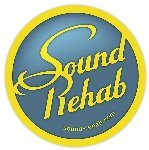Max Output Level: -47 dBFS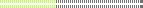• Total Posts : 2819
• Joined: 2011/02/03 04:31:35
• Location: Sound-Rehab, Austria
• Status: offlineRe: Behringer Deepmind 12 2018/11/06 08:55:41 (permalink)
Thanks. Will demo a DM12 soon, so this comes in handy :-)

But if I keep it and use it also with Studio One, this will be a long copy/paste thing to setup as S1 doesn't allow for loading such definitions (or at least I haven't found it yet?)

GOOD TUNES LAST FOREVER
+++   Visit the Rehab   +++

DAW: Platinum/X3e, win10 64 bit, i7-3930K (6x3.2GHz), Asus Sabertooth X79, 32 GB DDR3 1600MHz, ATI HD 5450, 120 GB SSD OCZ Agility3, 2x 1TB WD HDD SATA 600
Audio-Interface: 2x MOTU 1248 AVB, Focusrite OctoPre, (Roland Octa-Capture)   Control-Surface: VS-700C
VSTi: WAVES, NI K10u, FabFilter, IK, ... (too many really)
Bajan BlueMax Output Level: -55 dBFS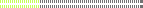• Total Posts : 2029
• Joined: 2005/09/15 20:54:56
• Location: Barbados & Cape Town
• Status: offlineRe: Behringer Deepmind 12 2018/11/10 15:22:00 (permalink)
Hi
Has anyone got and used a DeepMind 12 - I am a sucker for hardware synths, but tend to use older ones - but I would be interested to hear how Behringer stack up against say a latest Roland or Yamaha etc
Thanks
Nigel

Nigel
Check out the first single from our new Album, On Top of the World
https://www.youtube.com/watch?v=PTLVCOeTcTc

Check out our latest videos on
http://www.youtube.com/user/DevineLie/videos

Check out our website
www.devinelie.com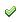Jump to:
© 2019 APG vNext Commercial Version 5.1